Home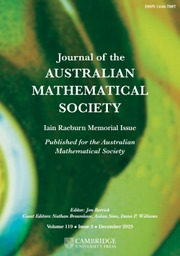Journal of the Australian Mathematical Society

# A ZERO-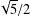$\!\sqrt{5}/2$ LAW FOR COSINE FAMILIES

Published online by Cambridge University Press:  17 April 2017

Corresponding

## Abstract

Let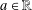$a\in \mathbb{R}$ , and let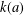$k(a)$ be the largest constant such that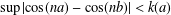$\sup |\text{cos}(na)-\cos (nb)|<k(a)$ for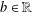$b\in \mathbb{R}$ implies that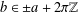$b\in \pm a+2\unicode[STIX]{x1D70B}\mathbb{Z}$ . We show that if a cosine sequence$(C(n))_{n\in \mathbb{Z}}$ with values in a Banach algebra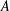$A$ satisfies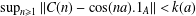$\sup _{n\geq 1}\Vert C(n)-\cos (na).1_{A}\Vert <k(a)$ , then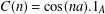$C(n)=\cos (na).1_{A}$ for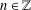$n\in \mathbb{Z}$ . Since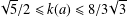$\!\sqrt{5}/2\leq k(a)\leq 8/3\!\sqrt{3}$ for every$a\in \mathbb{R}$ , this shows that if some cosine family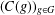$(C(g))_{g\in G}$ over an abelian group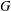$G$ in a Banach algebra satisfies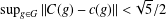$\sup _{g\in G}\Vert C(g)-c(g)\Vert <\!\sqrt{5}/2$ for some scalar cosine family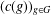$(c(g))_{g\in G}$ , then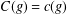$C(g)=c(g)$ for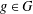$g\in G$ , and the constant$\!\sqrt{5}/2$ is optimal. We also describe the set of all real numbers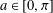$a\in [0,\unicode[STIX]{x1D70B}]$ satisfying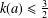$k(a)\leq \frac{3}{2}$ .

## MSC classification

Type
Research Article
Information
© 2017 Australian Mathematical Publishing Association Inc.

## Access options

Get access to the full version of this content by using one of the access options below.

## References

Arendt, W., Batty, C. J. K., Hieber, M. and Neubrander, F., Vector-Valued Laplace Transforms and Cauchy Problems (Birkhäuser, Basel, 2001).CrossRefGoogle Scholar
Arkhangel’skii, A. V. and Ponomarev, V. I., Fundamentals of General Topology: Problems and Exercises (D. Reidel Publishing Company/Hindustan Publishing Company, 1984), (translated from Russian).Google Scholar
Batkai, A., Engel, K.-J. and Haase, M., ‘Cosine families generated by second order differential operators on W 1, 1(0, 1) with generalized Wentzell boundary conditions’, Appl. Anal. 84 (2005), 867876.CrossRefGoogle Scholar
Bobrowski, A. and Chojnacki, W., ‘Isolated points of some sets of bounded cosine families, bounded semigroups, and bounded groups on a Banach space’, Studia Math. 217 (2013), 219241.CrossRefGoogle Scholar
Bobrowski, A., Chojnacki, W. and Gregosiewicz, A., ‘On close-to-scalar one-parameter cosine families’, J. Math. Anal. Appl. 429 (2015), 383394.CrossRefGoogle Scholar
Chojnacki, W., ‘On cosine families close to scalar cosine families’, J. Aust. Math. Soc. 99 (2015), 166174.CrossRefGoogle Scholar
Cox, R. H., ‘Matrices all of whose powers lie close to the identity’, Amer. Math. Monthly 73 (1966), 813.Google Scholar
Dales, H. G., Banach Algebras and Automatic Continuity, London Mathematical Society Monographs, 24 (Clarendon Press, Oxford, 2001).Google Scholar
Esterle, J., ‘Bounded cosine functions close to continuous scalar bounded cosine functions’, Int. Eq. Op. Th. 85 (2016), 347357.CrossRefGoogle Scholar
Haase, M., ‘The group reduction for bounded cosine functions on UMD spaces’, Math. Z. 262(2) (2009), 281299.CrossRefGoogle Scholar
Haase, M., ‘The functional calculus approach to cosine operator functions’, in: Recent Trends in Analysis, Proceedings of the Conference in honour of N. K. Nikolski held in Bordeaux 2011 (Theta Foundation, Bucharest, 2013), 123147.Google Scholar
Hirschfeld, R. A., ‘On semi-groups in Banach algebras close to the identity’, Proc. Japan Acad. Ser. A Math. Sci. 44 (1968), 755.Google Scholar
Kahane, J. P. and Salem, R., Ensembles Parfaits et Séries Trigonométriques (Hermann, Paris, 1963).Google Scholar
Nagy, B., ‘Cosine operator functions and the abstract Cauchy problem’, Period. Math. Hungar. 7 (1976), 1518.CrossRefGoogle Scholar
Nakamura, M. and Yoshida, M., ‘On a generalization of a theorem of Cox’, Proc. Japan Acad. Ser. A Math. Sci. 43 (1967), 108110.Google Scholar
Schwenninger, F. and Zwart, H., ‘Less than one, implies zero’, Studia Math. 229 (2015), 181188.Google Scholar
Schwenninger, F. and Zwart, H., ‘Zero-two law for cosine families’, J. Evol. Equ. 15 (2015), 559569.CrossRefGoogle Scholar
Travis, C. and Webb, G., ‘Cosine families and abstract nonlinear second order differential equation’, Acta Math. Acad. Sci. Hungar. 32 (1978), 7596.CrossRefGoogle Scholar
Wallen, L. J., ‘On the magnitude of ∥x n - 1∥ in a normed algebra’, Proc. Amer. Math. Soc. 18 (1967), 956.Google Scholar
Washington, L. C., Cyclotomic Fields, 2nd edn, Graduate Texts in Mathematics, 83 (Springer, New York–Berlin–Heidelberg, 1997).CrossRefGoogle Scholar

### Full text views

Full text views reflects PDF downloads, PDFs sent to Google Drive, Dropbox and Kindle and HTML full text views.

Total number of HTML views: 0
Total number of PDF views: 86 *
View data table for this chart

* Views captured on Cambridge Core between 17th April 2017 - 2nd December 2020. This data will be updated every 24 hours.

Hostname: page-component-79f79cbf67-t2s8l Total loading time: 2.58 Render date: 2020-12-02T07:56:38.417Z Query parameters: { "hasAccess": "0", "openAccess": "0", "isLogged": "0", "lang": "en" } Feature Flags last update: Wed Dec 02 2020 07:05:19 GMT+0000 (Coordinated Universal Time) Feature Flags: { "metrics": true, "metricsAbstractViews": false, "peerReview": true, "crossMark": true, "comments": true, "relatedCommentaries": true, "subject": true, "clr": false, "languageSwitch": true }

# Send article to Kindle

Note you can select to send to either the @free.kindle.com or @kindle.com variations. ‘@free.kindle.com’ emails are free but can only be sent to your device when it is connected to wi-fi. ‘@kindle.com’ emails can be delivered even when you are not connected to wi-fi, but note that service fees apply.

Find out more about the Kindle Personal Document Service.

A ZERO-$\!\sqrt{5}/2$LAW FOR COSINE FAMILIES Available formats × # Send article to Dropbox To send this article to your Dropbox account, please select one or more formats and confirm that you agree to abide by our usage policies. If this is the first time you use this feature, you will be asked to authorise Cambridge Core to connect with your <service> account. Find out more about sending content to Dropbox. A ZERO-$\!\sqrt{5}/2$ LAW FOR COSINE FAMILIES
Available formats
×

# Send article to Google Drive

To send this article to your Google Drive account, please select one or more formats and confirm that you agree to abide by our usage policies. If this is the first time you use this feature, you will be asked to authorise Cambridge Core to connect with your <service> account. Find out more about sending content to Google Drive.

A ZERO-$\!\sqrt{5}/2\$ LAW FOR COSINE FAMILIES
Available formats
×
×